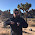## Sunday, July 17, 2016

### An ensemble of labor markets

Believe it or not, this post is actually a response of sorts to David Glasner's nice post "What's Wrong with Econ 101" and related to John Handley's request on Twitter for a computation of the implied unemployment rate given an output gap (which I will answer more directly as soon as I find the code that generated the original graph in question). It is also a "new" model, but still fairly stylized. I will start with the partition function approach described in the paper as well as here. However instead of being written in terms of money, I will write it in terms of labor.

Consider aggregate demand as a set of markets with demand $A = \{ a_{i} \}$, labor supply $L = \{ \ell_{i} \}$, information transfer indices (which I will label 'productivity states' for reasons that will become clear later) $p = \{ p_{i} \}$, and a price level $CPI = \{ cpi_{i} \}$. I use the notation:

$$cpi_{i} : a_{i} \rightleftarrows \ell_{i}$$

which just represents the differential equation (information equilibrium condition)

$$cpi_{i} \equiv \frac{da_{i}}{d\ell_{i}} = p_{i} \; \frac{a_{i}}{\ell_{i}}$$

This has solution

$$a_{i} \sim \ell_{i}^{p_{i}}$$

You can see how the $p_{i}$ values are related to 'productivity'; if the growth rate of $\ell_{i}$ is $r_{i}$, then the growth rate of $a_{i}$ is $p_{i} r_{i}$ and the ratio of $\ell_{i}$ to $a_{i}$ (assuming exponential growth for the two variables) is $e^{p_{i}}$.  Let's take the labor market to be a single aggregate (analogously to taking the money supply to be a single aggregate), so that we can drop the subscripts on the $\ell$'s. Define the partition function

$$Z(\ell) \equiv \sum_{i} e^{-p_{i} \log \ell}$$

so that the ensemble average of operator $X$ (which will be over 100 markets, i.e. $i = 1 .. 100$ with different values of $p_{i}$ in the Monte Carlo simulations below) is:

$$\langle X \rangle = \frac{\sum_{i} X \; e^{-p_{i} \log \ell}}{Z(\ell)}$$

I assumed the productivity states had a normal distribution which results in the following plot of 30 Monte Carlo throws for 100 markets for $\langle \ell^{p}\rangle$:

The ensemble of 'productivity states' $p_{i}$ looks something like this:

This picture illustrates David Glasner's point about general and partial equilibrium analysis in economics 101. The distribution (in black) represents a macroeconomic general equilibrium. Individual firms or markets will move from different productivity states over time. Partial equilibrium analysis looks at individual states that move or have idiosyncratic shocks that do not change the overall distribution. If the distribution changes, you have moved to a different general equilibrium and partial equilibrium analysis will not suffice. That is to say Economics 101 assumes this distribution does not change. (In this model, shocks are represented as productivity shocks, changing the values of $p_{i}$ and/or the average of the distribution in the figure.)

We can fit this ensemble average to the graph of nominal output (NGDP) versus total employees (PAYEMS on FRED, measured in Megajobs [Mj]):

The price level should then be given by the ensemble average $\langle p \ell^{p-1}\rangle$ (switching to linear scale instead of log scale):

The black lines in these graphs represent a single macroeconomic general equilibrium; as you can see, the true economy (blue) fluctuates around that equilibrium. Now the ensemble of individual markets can be represented as a single macro market, but with a (slowly) changing value of the single 'macro' information transfer index $p = \langle p_{i} \rangle$ plotted below:

Note that as the size of the labor market grows, productivity falls. This is analogous to the interpretation of the result for nominal output in terms of money supply: a large economy has more ways of being organized as many low growth states than as a few high growth ones. In thermodynamics, we'd interpret a larger economy (larger money supply or larger labor supply) as a colder economy.

The single macro market with changing $p$ is $CPI : A \rightleftarrows L$, which is basically the information equilibrium relationship that leads to Okun's law (shown in the paper as well as here in terms of hours worked, but labor supply also leads to a decent model, graph from here):

This correspondence means that we should view the difference between equilibrium output and actual output as well as the difference between the equilibrium price level and the actual price level as a measure of the output gap (difference from potential NGDP) and the unemployment rate, respectively ... which works pretty well for such a simple model:

Differences between the model and data could be interpreted as non-ideal information transfer which includes correlations among the labor markets (e.g. coordinating plans that fail together) or deviation from the equilibrium distribution of productivity states. Note that in the output gap calculation, you can see what looks like a plucking model of non-ideal information transfer with a changing equilibrium.

This simple model brings together output and employment, falling inflation and productivity, as well as macroeconomic general equilibrium and microeconomic partial equilibrium in a single coherent framework. It's not perfect empirically, but there isn't much competition out there!

...

Update 19 July 2016

Here's how the distribution of 'productivity' states changes as $\ell$ increases:

1.Good stuff! In your last graph you present the unemployment rate vs. model's perceived unemployment rate(?), which seems to fit very well since 1970. However, the decade or so before this it performs poorly compared to the actual data. Why might this be? End of Bretton Woods, perhaps?

1.I wouldn't necessarily ascribe it to any particular cause -- the model itself is a toy model in the sense of Narayana Kocherlakota's recent blog/white paper/essay.

My best guess is that it might have something to do with the employment population ratio changing, but there are so many things that would have to be done better to even start saying the model is wrong because of a particular effect rather than lack of attention to detail.

Also, the end of Bretton-Woods appears to have done nothing.